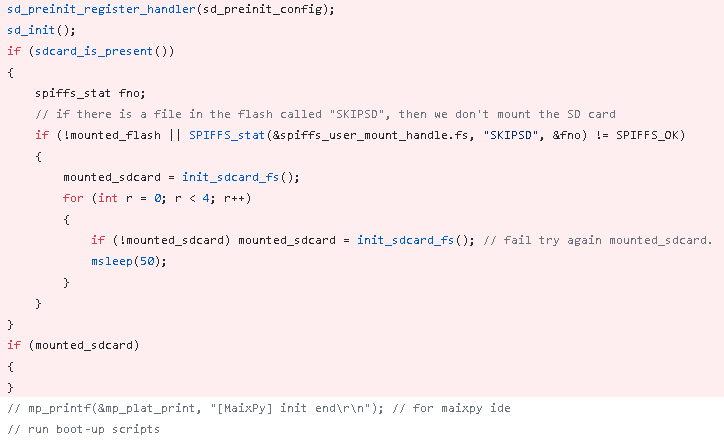# 以优化 MaixPy 的启动速度为例，说说 K210 的双核使用及原子操作。

## 文章大纲

• 优化 MaixPy 的 SD 卡挂载和启动问题。
• 示范一下双核的使用。
• 双核中需要的原子操作。

## MaixPy 进入系统很慢，慢在哪里了？

• 调用 maixpy_main 经过标准的 K210 BSP 启动过程，设置相应的芯片初始化，再配置 PLL \ RTC \ FLASH \ CORE 等硬件资源。

MaixPy/components/micropython/port/src/maixpy_main.c#L646-L703

• 再来进入到 mp_task 导入 MicroPython 的环境代码，选择执行的核心，并设置 Gc HeapSize 区域，它就类似于一个死循环，维持 MicroPython 的执行，在进入 REPL 之前，要完成 内存、外设、系统 的初始化。

MaixPy/components/micropython/port/src/maixpy_main.c#L488-L578

• 才到执行内置的 _boot.py 和 main.py 等内置代码。• sdcard_is_present 是为了确认 spi 与 sdcard 能够通信成功。
• init_sdcard_fs 是为了进一步初始化 SD 插入 MicroPython 环境中。

import esp

class FlashBdev:

SEC_SIZE = 4096
RESERVED_SECS = 1
START_SEC = esp.flash_user_start() // SEC_SIZE + RESERVED_SECS
NUM_BLK = 0x6b - RESERVED_SECS

def __init__(self, blocks=NUM_BLK):
self.blocks = blocks

#print("readblocks(%s, %x(%d))" % (n, id(buf), len(buf)))
esp.flash_read((n + self.START_SEC) * self.SEC_SIZE, buf)

def writeblocks(self, n, buf):
#print("writeblocks(%s, %x(%d))" % (n, id(buf), len(buf)))
#assert len(buf) <= self.SEC_SIZE, len(buf)
esp.flash_erase(n + self.START_SEC)
esp.flash_write((n + self.START_SEC) * self.SEC_SIZE, buf)

def ioctl(self, op, arg):
#print("ioctl(%d, %r)" % (op, arg))
if op == 4:  # BP_IOCTL_SEC_COUNT
return self.blocks
if op == 5:  # BP_IOCTL_SEC_SIZE
return self.SEC_SIZE

bdev = FlashBdev((size - 20480) // FlashBdev.SEC_SIZE - FlashBdev.START_SEC)MaixPy/commit/450f20b9956d88107109499a7eabfaf24021f4ed

## 双核要如何使用？

K210 的双核使用相关的 API 示范在 bsp 的 kendryte-standalone-sdk/lib/bsp/entry_user.ckendryte-standalone-sdk/lib/bsp/include/entry.h


typedef int (*dual_func_t)(int);
corelock_t lock;
volatile dual_func_t dual_func = 0;
void *arg_list;

{
while (1)
{
if (dual_func)
{ //corelock_lock(&lock);
(*dual_func)(1);
dual_func = 0;
//corelock_unlock(&lock);
}

//usleep(1);
}
}
int core1_function(void *ctx)
{
return 0;
}



dual_func = sd_preload; // int sd_preload(int core)


## 使用双核出现了问题？

    g_pixs_draw_pic_half_size = g_pixs_draw_pic_size/2;
g_pixs_draw_pic_half_size = (g_pixs_draw_pic_half_size%2) ? (g_pixs_draw_pic_half_size+1) : g_pixs_draw_pic_half_size;
g_pixs_draw_pic = p+g_pixs_draw_pic_half_size;

dual_func = swap_pixs_half; // 注册函数

for(i=0; i< g_pixs_draw_pic_half_size; i+=2)
{
#if LCD_SWAP_COLOR_BYTES
g_lcd_display_buff[i] = SWAP_16(*(p+1));
g_lcd_display_buff[i+1] = SWAP_16(*(p));
#else
g_lcd_display_buff[i] = *(p+1);
g_lcd_display_buff[i+1] = *p;
#endif
p+=2;
}

while(dual_func){} // 等待注册的函数执行完成


        if (maixpy_sdcard_loading) {
for(i=0; i< g_pixs_draw_pic_size; i+=2)
{
#if LCD_SWAP_COLOR_BYTES
g_lcd_display_buff[i] = SWAP_16(*(p+1));
g_lcd_display_buff[i+1] = SWAP_16(*(p));
#else
g_lcd_display_buff[i] = *(p+1);
g_lcd_display_buff[i+1] = *p;
#endif
p+=2;
}
} else {


## 原子操作要如何使用？


#include "atomic.h"

spinlock_t lock = SPINLOCK_INIT;

int set_call_back() {
spinlock_lock(&lock);

spinlock_unlock(&lock);
}



• 试图获取锁，如果失败则退出，确保了双核在并行执行时调取互斥资源的时候不会冲突。
      spinlock_lock(&lock);
spinlock_unlock(&lock);

• 试图设置变量到目标值，如果变量持续不满足则失败，类似 CAS 操作。
    int var = 0;


## 后记


def loop():

if state si 0:
pass
if state is 1:
pass


junhuanchen 2020年10月5日

posted @ 2020-10-05 19:13  Juwan  阅读(1222)  评论(0编辑  收藏  举报